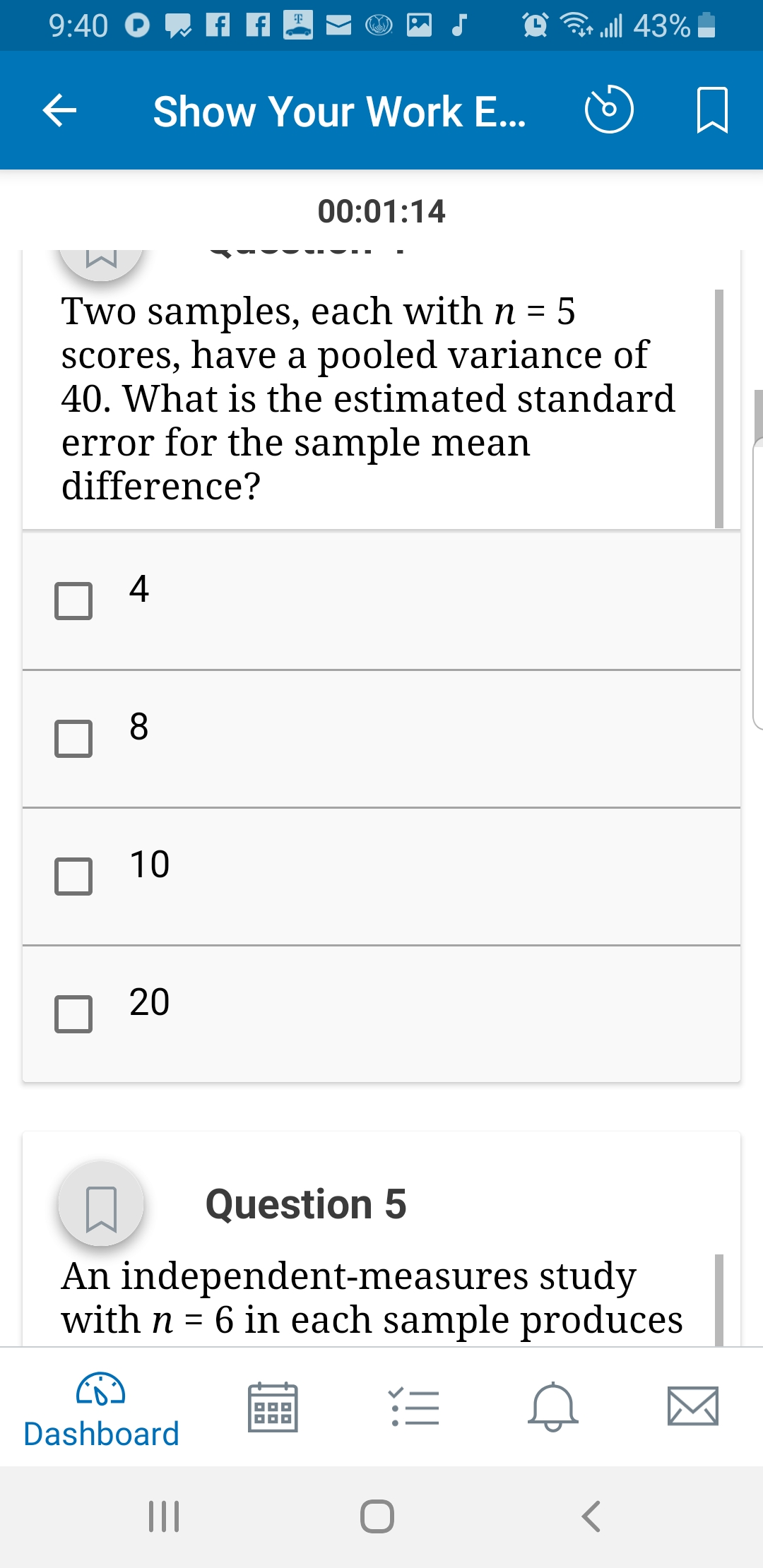# Show Your work E口00:01:14Two samples, each with n = 5scores, have a pooled variance of40. What is the estimated standarderror for the sample meandifference?41020Question 5An independent-measures studywith n = 6 in each sample producesDashboard

Question
53 viewshelp_outlineImage TranscriptioncloseShow Your work E 口 00:01:14 Two samples, each with n = 5 scores, have a pooled variance of 40. What is the estimated standard error for the sample mean difference? 4 10 20 Question 5 An independent-measures study with n = 6 in each sample produces Dashboard fullscreen
check_circle

Given pooled variance =Sp2 =  40

sample size of two samples = 5 so n1 = 5 ,  n2 = 5

The formula to calculate the standard error for sample mean dif...

### Want to see the full answer?

See Solution

#### Want to see this answer and more?

Solutions are written by subject experts who are available 24/7. Questions are typically answered within 1 hour.*

See Solution
*Response times may vary by subject and question.
Tagged in

### Measures